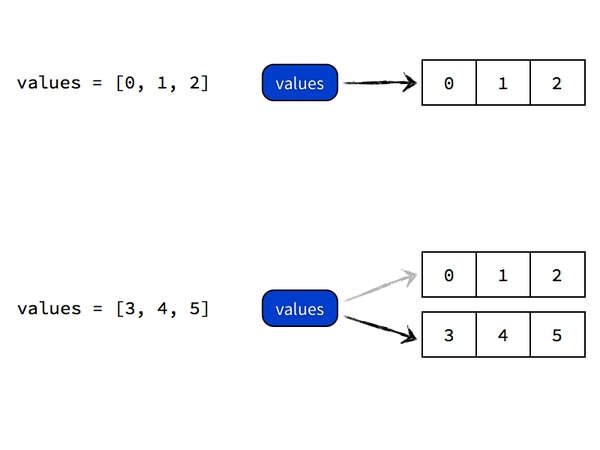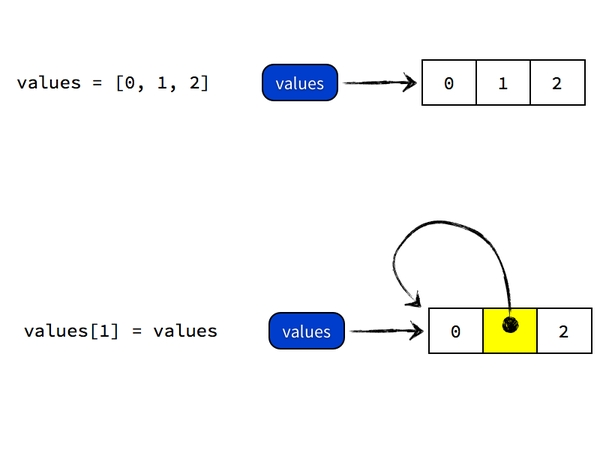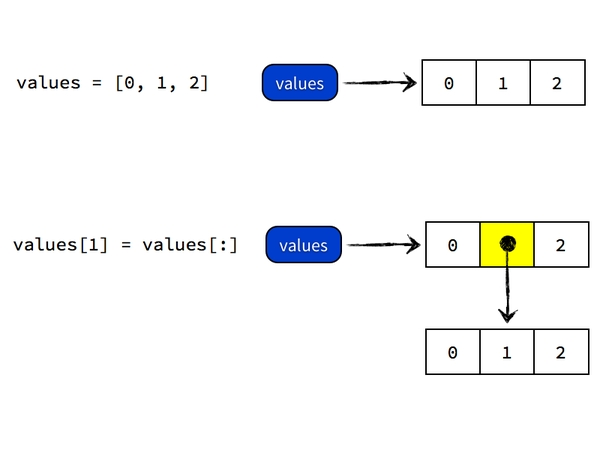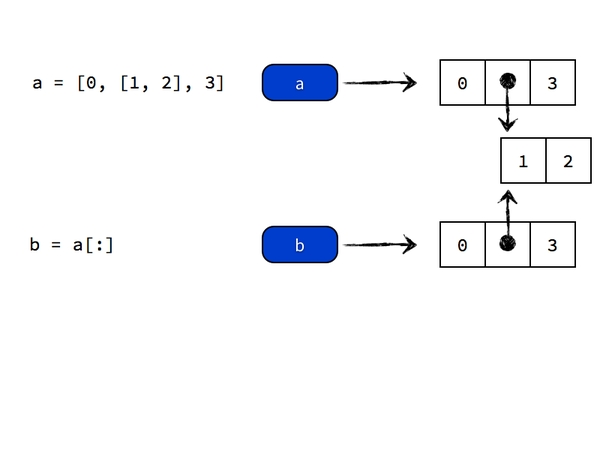# python基础（5）：深入理解 python 中的赋值、引用、拷贝、作用域

2014/03/03 10:18

### 1、先来看个问题吧：

 `1` `>>> values ``=` `[``0``, ``1``, ``2``]`
 `2` `>>> values[``1``] ``=` `values`
 `3` `>>> values`
 `4` `[``0``, [...], ``2``]`

``[0, [0, 1, 2], 2]``

Python 没有「变量」，我们平时所说的变量其实只是「标签」，是引用。

``values = [0, 1, 2]``

``values = [3, 4, 5]````values = values````values[:] #生成对象的拷贝或者是复制序列，不再是引用和共享变量，但此法只能顶层复制``

``values = values[:]``

Python 做的事情是，先 dereference 得到 values 所指向的对象 [0, 1, 2]，然后执行 [0, 1, 2][:] 复制操作得到一个新的对象，内容也是 [0, 1, 2]，然后将 values 所指向的列表对象的第二个元素指向这个复制二来的列表对象，最终 values 指向的对象是 [0, [0, 1, 2], 2]。过程如图所示：`1` `a ``=` `[``0``, [``1``, ``2``], ``3``]`
 `2` `b ``=` `a[:]`
 `3` `a[``0``] ``=` `8`
 `4` `a[``1``][``1``] ``=` `9``1` `import` `copy`
 `2`
 `3` `a ``=` `[``0``, [``1``, ``2``], ``3``]`
 `4` `b ``=` `copy.deepcopy(a)`
 `5` `a[``0``] ``=` `8`
 `6` `a[``1``][``1``] ``=` `9`

### 2、引用 VS 拷贝：

（1）没有限制条件的分片表达式（L[:]）能够复制序列，但此法只能浅层复制

（2）字典 copy 方法，D.copy() 能够复制字典，但此法只能浅层复制

（3）有些内置函数，例如 list，能够生成拷贝 list(L)

（4）copy 标准库模块能够生成完整拷贝：deepcopy 本质上是递归 copy

（5）对于不可变对象和可变对象来说，浅复制都是复制的引用，只是因为复制不变对象和复制不变对象的引用是等效的（因为对象不可变，当改变时会新建对象重新赋值）。所以看起来浅复制只复制不可变对象（整数，实数，字符串等），对于可变对象，浅复制其实是创建了一个对于该对象的引用，也就是说只是给同一个对象贴上了另一个标签而已。

 `01` `L ``=` `[``1``, ``2``, ``3``]`
 `02` `D ``=` `{``'a'``:``1``, ``'b'``:``2``}`
 `03` `A ``=` `L[:]`
 `04` `B ``=` `D.copy()`
 `05` `print` `"L, D"`
 `06` `print`  `L, D`
 `07` `print` `"A, B"`
 `08` `print` `A, B`
 `09` `print` `"--------------------"`
 `10` `A[``1``] ``=` `'NI'`
 `11` `B[``'c'``] ``=` `'spam'`
 `12` `print` `"L, D"`
 `13` `print`  `L, D`
 `14` `print` `"A, B"`
 `15` `print` `A, B`
 `16`
 `17`
 `18` `L, D`
 `19` `[``1``, ``2``, ``3``] {``'a'``: ``1``, ``'b'``: ``2``}`
 `20` `A, B`
 `21` `[``1``, ``2``, ``3``] {``'a'``: ``1``, ``'b'``: ``2``}`
 `22` `-``-``-``-``-``-``-``-``-``-``-``-``-``-``-``-``-``-``-``-`
 `23` `L, D`
 `24` `[``1``, ``2``, ``3``] {``'a'``: ``1``, ``'b'``: ``2``}`
 `25` `A, B`
 `26` `[``1``, ``'NI'``, ``3``] {``'a'``: ``1``, ``'c'``: ``'spam'``, ``'b'``: ``2``}`

### 3、增强赋值以及共享引用：

x = x + y，x 出现两次，必须执行两次，性能不好，合并必须新建对象 x，然后复制两个列表合并

x += y，x 只出现一次，也只会计算一次，性能好，不生成新对象，只在内存块末尾增加元素。

 `01` `L ``=` `[``1``, ``2``]`
 `02` `M ``=` `L`
 `03` `L ``=` `L ``+` `[``3``, ``4``]`
 `04` `print` `L, M`
 `05` `print` `"-------------------"`
 `06` `L ``=` `[``1``, ``2``]`
 `07` `M ``=` `L`
 `08` `L ``+``=` `[``3``, ``4``]`
 `09` `print` `L, M`
 `10`
 `11`
 `12` `[``1``, ``2``, ``3``, ``4``] [``1``, ``2``]`
 `13` `-``-``-``-``-``-``-``-``-``-``-``-``-``-``-``-``-``-``-`
 `14` `[``1``, ``2``, ``3``, ``4``] [``1``, ``2``, ``3``, ``4``]`

### 4、python 从 2k 到 3k，语句变函数引发的变量作用域问题

 `1` `def` `test():`
 `2` `    ``a ``=` `False`
 `3` `    ``exec` `(``"a = True"``)`
 `4` `    ``print` `(``"a = "``, a)`
 `5` `test()`
 `6`
 `7` `b ``=` `False`
 `8` `exec` `(``"b = True"``)`
 `9` `print` `(``"b = "``, b)`

 `1` `2K``：`
 `2` `a ``=`  `True`
 `3` `b ``=`  `True`
 `4`
 `5` `3K``：`
 `6` `a ``=`  `False`
 `7` `b ``=`  `True`

 `01` `def` `test():`
 `02` `    ``a ``=` `False`
 `03` `    ``ldict ``=` `locals``()`
 `04` `    ``exec``(``"a=True"``,``globals``(),ldict)`
 `05` `    ``a ``=` `ldict[``'a'``]`
 `06` `    ``print``(a)`
 `07`
 `08` `test()`
 `09`
 `10` `b ``=` `False`
 `11` `exec``(``"b = True"``, ``globals``())`
 `12` `print``(``"b = "``, b)`

http://stackoverflow.com/questions/7668724/variables-declared-in-execed-code-dont-become-local-in-python-3-documentatio

http://bugs.python.org/issue4831

http://stackoverflow.com/questions/1463306/how-does-exec-work-with-locals

http://woodpecker.org.cn/diveintopython3/porting-code-to-python-3-with-2to3.html

REF：

《learning python》：P130、P134、P202、P204 、P245

0
7 收藏

### 作者的其它热门文章0 评论
7 收藏
0